﻿ CONTROLLABILITY OF LINEAR FEEDBACK CONTROL SYSTEMS WITH COMMUNICATION CONSTRAINS
 林业科学2003, Vol. 39Issue (6): 131-135PDF
0

#### 文章信息

Zhou Yucheng, Cheng Fang, An Yuan, Chen Yongping, Zhao Hui

CONTROLLABILITY OF LINEAR FEEDBACK CONTROL SYSTEMS WITH COMMUNICATION CONSTRAINS

Scientia Silvae Sinicae, 2003, 39(6): 131-135.

### 作者相关文章

CONTROLLABILITY OF LINEAR FEEDBACK CONTROL SYSTEMS WITH COMMUNICATION CONSTRAINS
Zhou Yucheng, Cheng Fang, An Yuan, Chen Yongping, Zhao Hui
Research Institute of Wood Industry, CAF Beijing 100091
Abstract: In wood industry engineering systems, the observation vector is often not available in its entirety to the controller, but a quantized version of it transmitted over a communication channel with accompanying transmission delays and distortion, subject to bit rate constraints. This problem has attracted some attention and progress is little in recent years. Feedback control problems for a linear continuous-time system with communication constraints are considered in this paper. The concept of set-controllability is introduced and testified for such a system. Moreover, sufficient and necessary conditions are provided for the systems' controllability, then the system can be reaching.
Key words: Wood industry engineering systems    Linear systems    Controllability
1 Introduction

In many real engineering systems, the observation vector is often not available in its entirety to the controller(Borker et al., 1996; Caines et al., 1996; Cover et al., 1991), but a quantized version of it transmitted over a communication channel with accompanying transmission delays and distortion, subject to bit rate constraints. This problem has attracted some attention in recent years, see, e.g. (Delchamps, 1990).

The aim of this note is to study the impact of the communication constraints on properties of linear feedback control systems. The model studied here consists of a plant and a controller which locate far from each other. The observation of the plant is quantized, coded and transmitted to the remote controller via a communication channel with finite bandwidth. Once the coded observation is received by the controller, it is decoded and the feedback control is computed and decoded, and transmitted back to the plant. Obviously, the system can never be asymptotically stabilized if the uncontrolled dynamics is unstable due to the inherent delay in the feedback control caused by the finite communication capacity. So a new concept called containability is introduced by Wong and Brockett(1995).They also provided necessary conditions as well sufficient ones for systems to be containable. On the other hand, the concept of usual controllability is no longer meaningful in this case because the information about state of the system is quantized measurements instead of exact measurements. Instead, a weaker controllability concept, called set-controllability is introduced. Some necessary and sufficient conditions for systems to be set-controllable are derived in the note. The relationship between the containability and controllability will be addressed.

The note is organized as follows. Section 2 formulates control problems with communication constraints for linear systems. In Section 3, some definitions are introduced, and some sufficient conditions for systems to be controllable are derived. The conclusion is given in Section 4.

2 Control problem with communication constraints

Consider a continuous-time system with linear dynamics: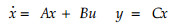(1)

where xRn, uRm, and yRr, A, B and C are matrices with appropriate dimensions.

The observation of x(t), y(t) is transmitted to a remote decision-maker for computing the appropriate level of feedback control. For simplicity, assume that it takes δ=1/R second to send one bit from the plant to the controller and visa versa from the controller to the plant, where R is the transmission rate in bits per second. Hence, if a bit is sent at time zero, it will be received at time δ at the receiver. Unlike classical models, the observed information is not transmitted continuously. Therefore, we assume that x(t) is sampled at time instants {ri}i=0 with r0=0; the other sample instants will be defined later.

Before an observation can be transmitted, it must be quantized and coded for the transmission. It is assumed that prefix codes are used so that the ending of a codeword is immediately recognizable(Cover et al., 1991).The quantization and coding function can be symbolically represented by a function H from the state space Rn to D where D stands for the set of finite length strings of symbols from a D-ary symbol set. Therefore, the i-th transmitted codeword from the plant to the controller is ci=H[y(ri)].

Let l denote the codeword length function. Then the codeword is received by the controller at time si=ri+l(ci)δ. Once the coded observation is received, it is decoded and the feedback control is computed and coded for transmission back to the plant. Assume the Markovian feedback law(Wong et al., 1995)is used, that is, the i-th control codeword is di=g(ci), where g is function from D to Rm. It is easily seen that the control codeword di reaches at the plant at time ti=ri+l(ci)δ+l(di)δ. We assume an impulse control scheme is used in the sense that: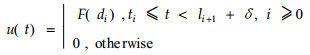In summary, the Markovian Finite Communication Control (MFCC) model can be expressed by the following set of equations: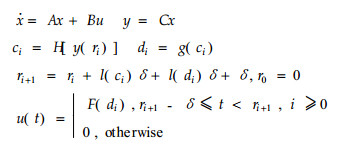(2)

A MFCC system with unstable modes can not be made asymptotically stable since there is the delay between observation instants and control time(Wong et al., 1995). In this note, the study will be focused on a weaker controllability, namely set-controllability.

3 Definitions

For the system(2), the concept of controllability is no longer meaningful for lack of information of every point. Therefore, in what follows, we will introduce the notion of set-controllability for(2).

Definition 1 (Partition) (Caines et al., 1996) A finite analytic partition of the state space DRn of (2) is a pairwise disjoint collection of subsets π={x1, x2, L, xnπ} such that each xi is path-connected and of non-zero Lebesgue measure, and is such that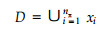Moreover, if for all i=1, …, nπ, xi is a rectangular cube whose edges are parallel to the coordinate axes and which has length Δi in the direction xj, j=1, …, n, then the partition π is called a uniform partition (Delchamps et al., 1990), denoted as πu. If Δ1=…=Δn=Δ, Δn=1, then the partition π is called an equal uniform partition, denoted as πue. If Δ1=…=Δn=1, then the partition π is called a unit uniform partition, denoted as πuμ.

Definition 2   For a given partition π, a bounded path-connected set M with non-aero Lebesgue measure is said to be controllable by one step to another bounded path-connected set N, also with non-zero Lebesgue measure, if for any non-empty set MjMπ there exists a corresponding coded feedback control uj such that all the points started in Mj enters N at the sampling instant r=[l(cj)+l(di)+1]δ where Mπ={Mxi, i=1, …, nπ}.

Let q denote the number of non-empty sets in Mπ, and M1, …, Mq, the q non-empty sets in Mπ.

Lemma 1 Assume that for all j=1, …, q, there exist a point xjMj and an input uj such that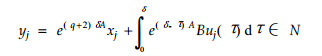and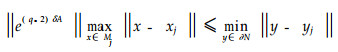with ∂ N the boundary of N. Then M is controllable by one step to N.

Proof: All the points observed in Mi are coded with the codeword ci with length i, and the feedback control, ui, corresponding to the codeword ci is coded by the codeword ei with length q-i+1. It follows that r=q+2. For brevity and convenience, such a coded feedback is referred as to standard coded feedback.

Let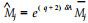, Then according to relation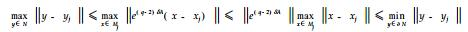(3)

It is easily seen that all the points started in Mj enters in N at r=q+2 for all j, which implies that. M is controllable by one step to N.

For the case that the system is controllable, the following corollary can be easily obtained.

Corollary 1 Assume that the system (1) is controllable. Then

For a given uniform partition πu, M is controllable by one step to N if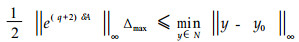with y0N and Δmax=max{Δi, i=1, l, n};

For a given equal uniform partition πue, M is controllable by one step to N if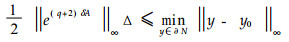For a given unit uniform partition πuu, M is controllable by one step to N if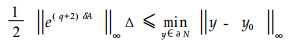Proof: Since the system is controllable, it follows that there exists uj such that the center of Mj can be steered to y0 for all 1≤jq. The results can be easily verified by using Lemma 1.

From Corollary 1, the following results can be easily proved.

Corollary 2 Assume that the system (1) is controllable. Then

For a given uniform partition πu, M is controllable by one step to a cube O with the length of side ro if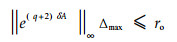For a given equal uniform partition πue, M is controllable by one step to a cube O with the length of side ro ifFor a given unit uniform partition πuu, M is controllable by one step to a cube O with the length of side ro if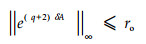If A is in a triangular form with maximal eigenvalue λmax, it is possible to prove the following theorem which provides sufficient and necessary conditions for a set, containing at least one set of πeu or πuu, to be controllable to a cube by one step and by using standard coded feedback.

Theorem 1 Assume that A is in a triangular form with maximal eigenvalue λmax, B and C are nonsingular and O is a cube with the length of side ro, and consider the standard coded feedback. Then

For a given equal uniform partition πeu, a set M, which contains at least one set of πue, is controllable by one step to O if and only if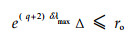For a given unit uniform partition πuu, a set M, which contains at least one set of πuu, is controllable by one step to O if and only if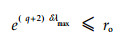Proof: The sufficiency is the straightforward consequence of Corollary 2. Now let us prove its necessity. If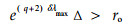then for XM⌒πue (there always exists such an X since M contains at least one set of πeu), it is easily seen that there are at least some points of the image e(q+2)δAX whose coordinate values in the direction associated with λmax are larger than ro. As a consequence, M can not be controllable by one step to O.

Note that some sets which can not be controlled by one step to a certain set may be controlled by several steps to the set. The following example is the case.

Example 1 Consider the system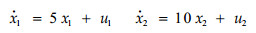Now assume that δ=0.009 and Δ=Δ12=1. Consider the sets M and N depicted, It is easily seen that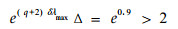which means that M can not be controlled by one step to N. Fortunately, a straightforward calculation shows that M can be controlled by one step to a rectangular, say L, whose sides have lengths of 3 and 2. Due to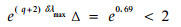(4)

it follows from Corollary L is controllable by one step to N. So we may say M is controllable by two steps to N.

Definition 3 For a given partition π, a bounded path-connected set M with non-zero Lebesgue measure is said to be controllable by p steps to another bounded path-connected set N, also with non-zero Lebesgue measure, if there exist p sets, say M1, …, Mp, such that Mi is controllable by one step to Mi+1 for all 0≤ip+1 with M0=M and Mp+1=N.

Theorem 2 Consider the following system: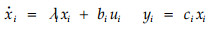(5)

with ai>0, bi and ci non-zero, i=1, …, n. Consider a unit uniform partition πuu. Assume that M={xRn:αixiβi, i=1, …, n} with βiαi+1 for all 1≤in, and N={xRn:γixiμi, i=1, …, n}. Then M is controllable by p steps to N if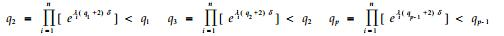(6)

and for all 1≤in,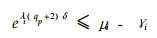(7)

with q1=∏i=1n[βi-αi], where [α] denotes the smallest integer larger than α.

Proof: Without loss of generality, assume that α1=α2=…=αn=0. It is obvious that there are q1 cubes in πuu which intersect with M. As a result, r=(q1+2)δ. It is easily seen that each cube can be controlled by one step via a standard coded feedback to the set: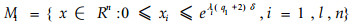Similarly, there are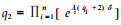cubes in πuu which intersect with M1. Thus, each cube of these q2 cubes can be steered by one step via a standard coded feedback to the set: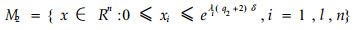By induction, the set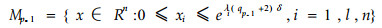can be driven by one step via a standard coded feedback to the set: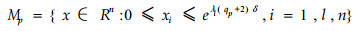which is contained in N due to (6).

4 Conclusion

The problem of feedback control for a linear system with finite communication capacity is discussed. The concept of set-controllability is introduced, and sufficient and necessary conditions are provided for a system to be set-controllable.

References
 Borker V S, Mitter S K. 1996. LQG control with communication constraints. Manuscript: 58-62. Caines P E, Wei Y J. 1996. Hierarchical hybrid control systems: a lattice theoretic formulation. Manuscript: 236-277. Cover T M, Thomas J A. 1991. Elements of information theory. New York: Wiley-Interscience Publication: 236-277. Delchamps D F. 1990. Stabilizing a linear system with quantized state feedback. IEEE Trans. Automatic Control, 35: 916-924. DOI:10.1109/9.58500 Kalamba P T, Hara S. 1993. Worst-case analysis and design of sampled-data control systems. IEEE Trans. Automatic Control, 38: 1337-1357. DOI:10.1109/9.237646 Kulkarni S R, Ramadge P J. 1996. Bit rate bounds for state tracking with finite rate communication. Proc. CDC: 47-59. Li X, Wong W S. 1997. State estimation with communication constraints. Systems & Control Letters: 743-762. Williamson D. 1985. Finite wordlength design of digital Kalman filters for state estimation. IEEE Trans. Automatic Control, 30: 930-939. DOI:10.1109/TAC.1985.1103826 Wong W S, Brockett R W. 1995. Systems with finite communication bandwidth constraints: feedback control problems. Proc. Of IEEE CDC: 876-886. Wong W S, Brockett R W. 1998. Systems with finite communication bandwidth constraints: state estimation problems. IEEE trans. Automatic Control: 1336-1347.